# Solved Problems on Semiconductor Diode

### Solution :

Peak input voltage = 20 V
Forward resistance, r= 10 Ω
Potential barrier voltage, V0 = 0.7 V
The diode will conduct during the positive half-cycles of a.c. input voltage only.

The equivalent circuit is shown in Fig.1(ii)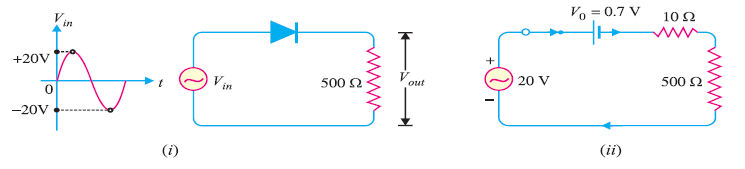Fig. 1

(i) The peak current through the diode will occur at the instant when the input voltage reaches positive peak i.e. Vin = VF = 20 V.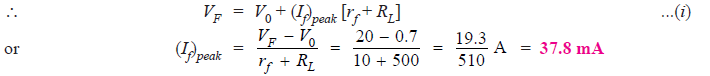(ii) Peak output voltage :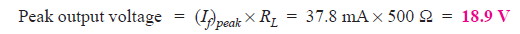Ideal Diode Case:

### Q2. Find the current through the diode in the circuit shown in Fig. 2(i). Assume the diode to be ideal.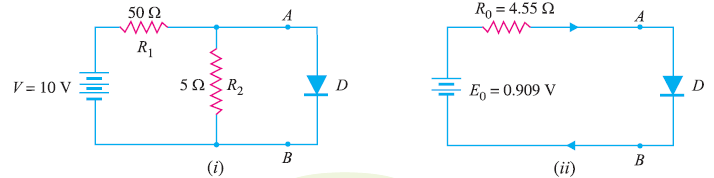Fig. 2

### Solution :

We shall use Thevenin’s theorem to find current in the diode. Referring to Fig. 2(i),Fig. 2 (ii) shows Thevenin’s equivalent circuit. Since the diode is ideal, it has zero resistance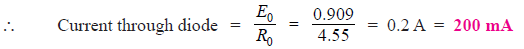### Q3. Calculate the current through 48 Ω resistor in the circuit shown in Fig. 3 (i). Assume the diodes to be of silicon and forward resistance of each diode is 1 Ω.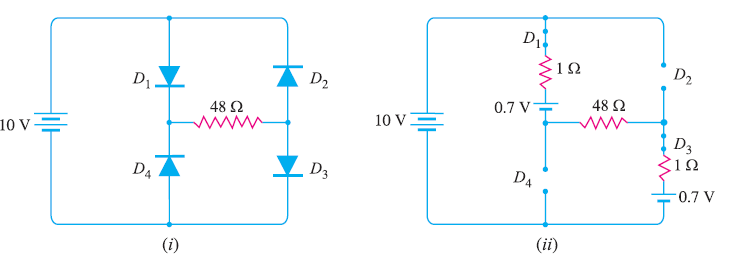Fig. 3

### Solution :

Diodes D1 and D3 are forward biased while diodes D2 and D4 are reverse biased. We can, therefore, consider the branches containing diodes D2 and D4 as “open”.

Replacing diodes D1 and D3 by their equivalent circuits and making the branches containing diodes D2 and D4 open, we get the circuit shown in Fig. 3 (ii). As we know for a silicon diode, the barrier voltage is 0.7 V.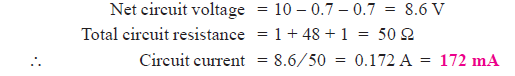### Q4. Determine the current I in the circuit shown in Fig. 4 (i). Assume the diodes to be of silicon and forward resistance of diodes to be zero.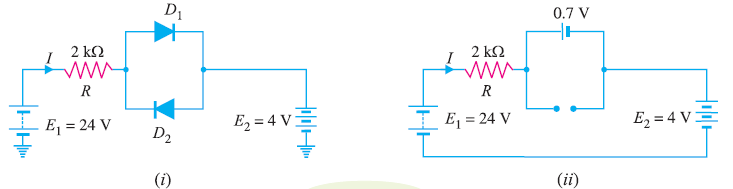Fig. 4

### Solution :

The conditions of the problem suggest that diode D1 is forward biased and diode D2 is reverse biased. We can, therefore, consider the branch containing diode D2 as open as shown in Fig. 4 (ii).

Further, diode D1 can be replaced by its simplified equivalent circuit.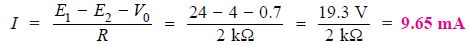### Q5. Find the voltage VA in the circuit shown in Fig. 5 (i). Use simplified model.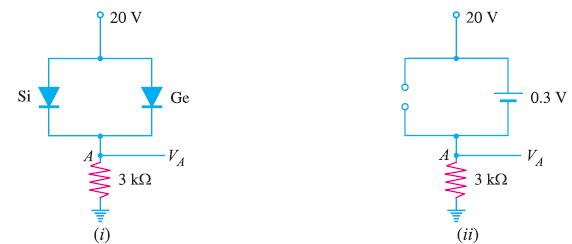Fig. 5

### Solution :

It appears that when the applied voltage is switched on, both the diodes will turn “on”. But that is not so. When voltage is applied, germanium diode (V0 = 0.3 V) will turn on first and a level of 0.3V is maintained across the parallel circuit.

The silicon diode never gets the opportunity to have 0.7 V across it and, therefore, remains in open-circuit state as shown in Fig.5(ii).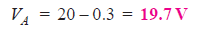### Q6. Find VQ and ID in the network shown in Fig. 6(i). Use simplified model.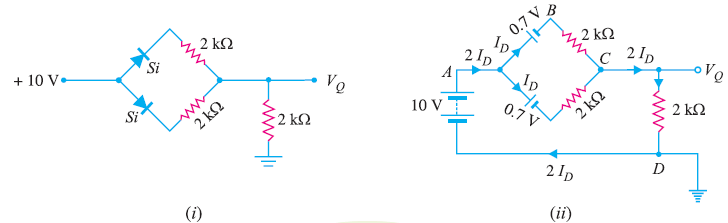Fig. 6

### Solution :

Replace the diodes by their simplified models. The resulting circuit will be as shown in Fig. 6 (ii).

By symmetry, current in each branch is Iso that current in branch CD is 2ID.

Applying Kirchhoff’s voltage law to the closed circuit ABCDA, we have,### Solution :

The applied voltage forward biases each diode so that they conduct current in the same direction. Fig. 7 (ii) shows the equivalent circuit using simplified model. Referring to Fig. 7 (ii),### Solution :

As we can see in Fig. 8 (i)  both diodes D1 and D2 are forward biased. Using simplified model for the diodes, the circuit shown in Fig. 8(i) becomes the one shown in Fig. 8 (ii).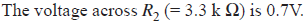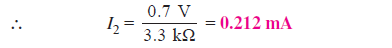Applying Kirchhoff’s voltage law to loop ABCDA in Fig. 8 (ii), we have,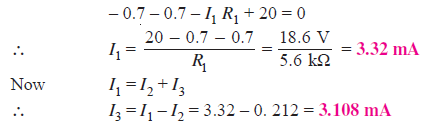### Q9. Determine if the diode (ideal) in Fig. 9 (i) is forward biased or reverse biased.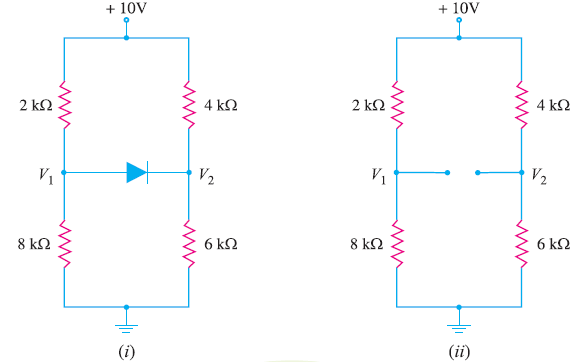Fig. 9

### Solution :

Let us assume that diode in Fig.9 (i) is OFF i.e. it is reverse biased.

The circuit then becomes as shown in Fig. 9(ii). Referring to Fig. 9 (ii), we have,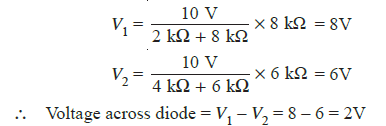Now V1 – V2 = 2V is enough voltage to make the diode forward biased. Therefore, our initial assumption was wrong, and diode is forward biased.

### Q10. Determine the state of diode for the circuit shown in Fig. 10 (i) and find ID and VD . Assume simplified model for the diode.Fig. 10

### Solution :

Let us assume that the diode is ON. Therefore, we can replace the diode with a 0.7V battery as shown in Fig. 10 (ii). Referring to Fig.10 (ii), we have,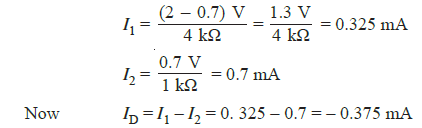Since the diode current is negative, the diode must be OFF and the true value of diode current is ID =0 mA. Hence our initial assumption was wrong.

In order to analyse the circuit properly, we should replace the diode in Fig. 10 (i) with an open circuit as shown in Fig.10(iii).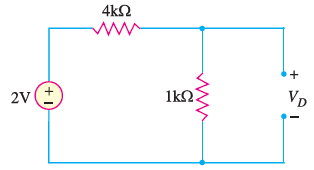Fig.10 (iii)

The voltage VD across the diode is :We know that 0.7V is required to turn ON the diode. Since VD is only 0.4V, the answer confirms that the diode is OFF.